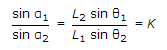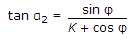# Civil Engineering - Docks and Harbours

### Exercise :: Docks and Harbours - Section 1

31.

Pick up the incorrect statement from the following: In a dry dock block made of hard wood,

 A. spacing of the blocks is 1.35 m B. the lowest block is 1.8 m long 40 cm x 40 cm in cross-section C. the middle block is 1.6 m long 40 cm x 40 cm in crosss-section D. the top block is 1.2 m long 40 cm x 40 cm in cross-section E. none of these.

Explanation:

No answer description available for this question. Let us discuss.

32.

On a hydrographic map, the following feature is shown :

 A. datum B. high and low water lines C. depth contours D. land and water areas E. all the above.

Explanation:

No answer description available for this question. Let us discuss.

33.

Due to the impact of water wave on a sea shore structure

 A. hydrostatic pressure coupled with a strong momentary impact is caused B. vibrations are subjected C. internal pressure is developed D. weakens the foundation E. all of the above.

Explanation:

No answer description available for this question. Let us discuss.

34.

The horizontal angles from the boat between A and B and B and C, the stations on the shore are respectively θ1 and θ2. The distances AB = L1 and BC = L2. For calculating the angle α2 at C between the boat and station B is obtained by using the following formula: where (β is horizontal angle between A and C at B).

 A.B. αt tan α2 = 360° - (θ1 + θ2 + β) = φ C.D. all the above.

Explanation:

No answer description available for this question. Let us discuss.

35.

Pick up the correct statement from the following:

 A. The breakwater which can be used as a platform for loading and unloading of cargo is called a mole B. The brick masonry retaining wall which is used for loading and unloading of cargo is called guay wall C. Three types of break waters are generally provided in harbours D. All of the above.